May 29, 2020

Remember Me

### Create an account

Fields marked with an asterisk (*) are required.
Name *
Email *
Verify email *## Clustering

ML Unsupervised

User Rating: 0 / 5This document contains a presentation of the clustering technique, we will start with its definition, then we will present the application domains. After that, we will see its main approaches, and we will detail just the partitioning approach which contains 2 algorithms: k-means and k-medoids.

• Definition
• Application domains
• Major Clustering Approaches
• K-means algorithm
• K-medoids algorithm

### 1- Definition:

Clustering can be considered the most important unsupervised learning problem; so, as every other problem of this kind, it deals with finding a structure in a collection of unlabeled data.
A loose definition of clustering could be “the process of organizing objects into groups whose members are similar in some way”.
cluster is therefore a collection of objects which are “similar” between them and are “dissimilar” to the objects belonging to other clusters.

### 2- Application domains:

Clustering algorithms can be applied in many fields, for instance:

• Marketing: finding groups of customers with similar behavior given a large database of customer data containing their properties and past buying records;
• Biology: classification of plants and animals given their features;
• Libraries: book ordering;
• Insurance: identifying groups of motor insurance policy holders with a high average claim cost; identifying frauds;
• City-planning: identifying groups of houses according to their house type, value and geographical location;
• Earthquake studies: clustering observed earthquake epicenters to identify dangerous zones;
• WWW: document classification; clustering weblog data to discover groups of similar access patterns.

### 3- Major Clustering Approaches:

• Hierarchical clustering: Create a hierarchical decomposition of the set of data (or objects) using some criterion
• Density-based clustering: based on connectivity and density functions
• Grid-based clustering: based on a multiple-level granularity structure
• Model-based clustering: A model is hypothesized for each of the clusters and the idea is to find the best fit of that model to each other
• Partitional clustering: Construct a partition of a database D of n objects into a set of k clusters, it’s divided into two main sub-categories: centroid-based algorithms and medoid-based algorithms.
• k-means(MacQueen’67): Each cluster is represented by the center of the cluster
• k-medoids or PAM (Partition around medoids) (Kaufman & Rousseeuw’87): Each cluster is represented by one of the objects in the cluster

### 4- K-means algorithm:

Principle of the algorithm:

K-means is an iterative clustering algorithm that aims to find local maxima in each iteration. This algorithm works in these 5 steps:

1. Specify the desired number of clusters K
2. Specify the centroids of each cluster
3. Compute the distance from the Centroids
4. Group objects based on the minimum distance
5. If there is a movement of the objects repeat steps 234! if not, we finish the algorithm and exit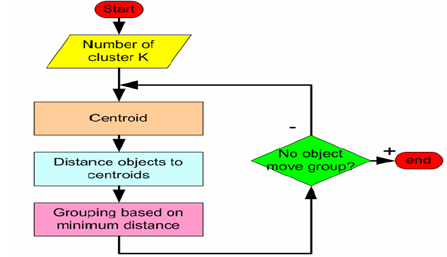Exercise:

Use the k-means algorithm and Euclidean distance to cluster the following 8 examples into 3 clusters: A1=(2,10), A2=(2,5), A3=(8,4), A4=(5,8), A5=(7,5), A6=(6,4), A7=(1,2), A8=(4,9). The distance matrix based on the Euclidean distance is given below: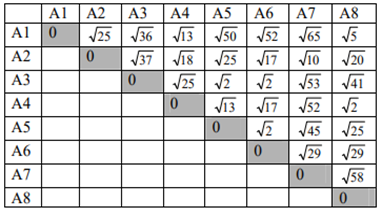Suppose that the initial seeds (centers of each cluster) are A1, A4 and A7. Run the k-means algorithm for 1 epoch only. At the end of this epoch show:

1. The new clusters (i.e. the examples belonging to each cluster)
2. The centers of the new clusters
3. Draw a 10 by 10 space with all the 8 points and show the clusters after the first epoch and the new centroids.
4. How many more iterations are needed to converge? Draw the result for each epoch.

Solution:

a) d(a,b) denotes the Euclidian distance between a and b. It is obtained directly from the distance matrix or calculated as follows: d(a,b)=sqrt((xb-xa)2 +(yb-ya)2 ))

seed1=A1=(2,10), seed2=A4=(5,8), seed3=A7=(1,2)

epoch1 – start: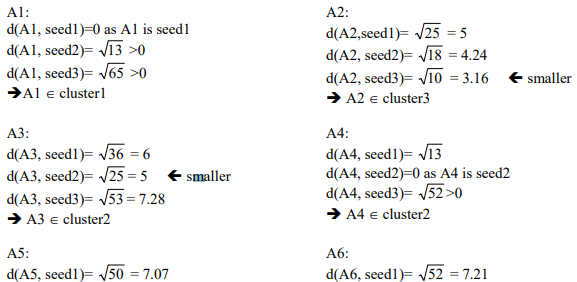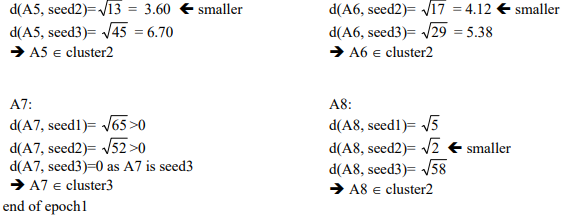New clusters: 1: {A1}, 2: {A3, A4, A5, A6, A8}, 3: {A2, A7}

b) The centers of the new clusters: C1= (2, 10), C2= ((8+5+7+6+4)/5, (4+8+5+4+9)/5) = (6, 6), C3= ((2+1)/2, (5+2)/2) = (1.5, 3.5)

c)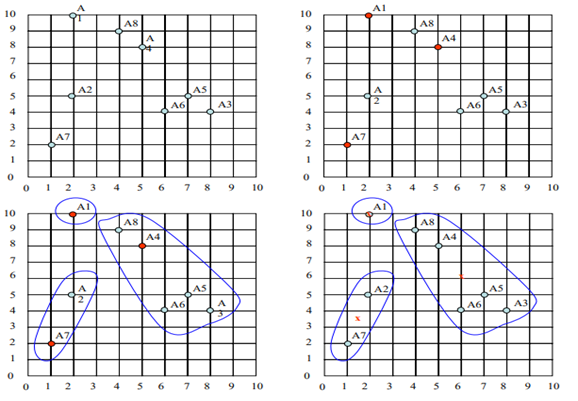d) We would need two more epochs. After the 2nd epoch the results would be:

1: {A1, A8}, 2: {A3, A4, A5, A6}, 3: {A2, A7} with centers C1=(3, 9.5), C2=(6.5, 5.25) and C3=(1.5, 3.5).

After the 3rd epoch, the results would be: 1: {A1, A4, A8}, 2: {A3, A5, A6}, 3: {A2, A7} with centers C1=(3.66, 9), C2=(7, 4.33) and C3=(1.5, 3.5).

• Easy to implement
• With a large number of variables, k-means may be computationally faster than hierarchical clustering (if k is small).
• K-means may produce tighter clusters than hierarchical clustering
• An instance, can change cluster (move to another cluster) when the centroids are recomputed.

• Difficult to predict the number of clusters (K-Value)
• Initial seeds have a strong impact on the final results
• The order of the data has an impact on the final results

### 5- K-medoïds algorithm:

· In the k-medoids approach, a cluster is represented by one of its points. This representative point is called a medoid, it is a point that is most placed at the center taking into account some measurements, such as distance.

· Several algorithms based on the notion of the medoid exist as PAM (Partitioning Around Medoids) which is a k-medoids algorithm that tries to group a set of m points into k clusters.

Principle of algorithm:

The PAM algorithm, proposed by kaufman and rousseuw in 1990, is based on the following principle:

Start with a set of medoids and then iteratively replace one with another if it reduces the overall distance.

• Use real object to represent the cluster
• Select k representative objects arbitrarily
• For each pair of non-selected object h and selected object i, calculate the total swapping cost TCih
• For each pair of i and h,
• If TCih < 0, i is replaced by h
• Then assign each non-selected object to the most similar representative object
• repeat steps 2-3 until there is no change

Example:

Assuming that in this example, our data set consists of 10 objects that are defined by 2 attributes X and Y, and that we want to partition these objects into 2 different clusters.

Step 1:

We will choose arbitrarily 2 medoids c1 = (3,4) and c2 = (7,4)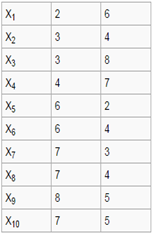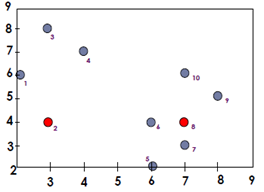We will calculate the distance between each object and the chosen medoid, in order to associate each object with the nearest medoid.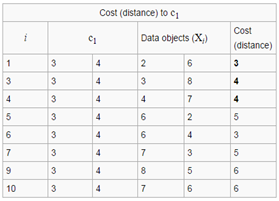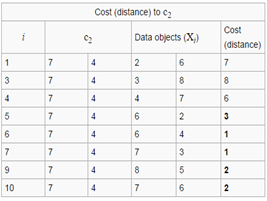The distance of Manhattan: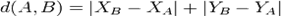Cluster1={X1,X2,X3,X4}

Cluster2={X5,X6,X7,X8,X9,X10}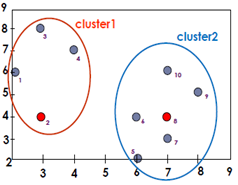Then we will calculate the total cost of this configuration, which is the sum of the distances between the objects and the medoid in each cluster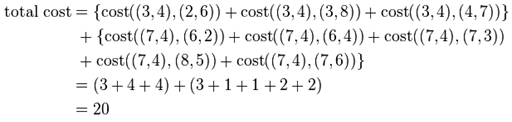Step 2: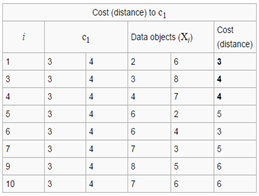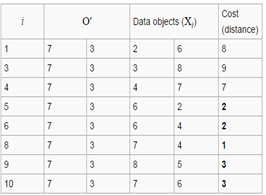The medoids now are: c1 (3.4) and O' (7.3)

total cost = 3+4+4+2+2+1+3+3

= 22

We will calculate the cost function between the 2 configurations.

S = current total cost – past total cost

= 22-20

= 2 > 0

These are the new clusters: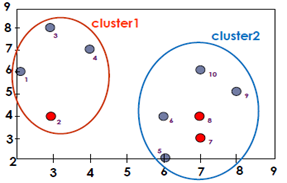Since it gives a positive value, it means that choosing the X7 object to be the medoid was a bad idea, so our first choice was good, but to decide whether the configuration is good or not, you have to try another non medoid object and if you find that the cost function remains positive, at that moment you can decide that the first choice was good, so the configuration does not change and the algorithm ends.

• K-medoids method is more robust than k-means in the presence of noise and outliers
• Flexible with any type of distance

• K-medoids is more costly that the k-means method
• Like k-means, k-medoids requires the user to specify k
• It does not scale well for large data sets

author:

Kawtar NAJMANI

PhD Condidat at faculty of science Ben M Sik.

## Analysis of Single-Layer Networks in Unsupervised Feature Learning

ML Unsupervised

User Rating: 0 / 5Much recent work in machine learning has focused on learning good feature representations from unlabeled input data for higher-level tasks such as classification.

## How does unsupervised machine learning work?

ML Unsupervised

User Rating: 0 / 5machine learning is something like a process of probability, where a machine build up experience from previous results of the same process and then react.

## Supervised vs. Unsupervised Machine Learning

ML Unsupervised

User Rating: 0 / 5Data scientists use many different kinds of machine learning algorithms to discover patterns in big data that lead to actionable insights

### Popular Articles#### IA&NLP’20: International Workshop on Artificia…

May 03, 2020 Category (en-us)

### Trending Now#### Scikit-learn: Machine Learning in Python

Apr 06, 2018 Rate: 0.00#### An Introduction to MCMC for Machine Lear…

Apr 06, 2018 Rate: 0.00#### Treillis de concepts et classification s…

Apr 08, 2018 Rate: 0.00